# Pythagoras Theorem

A Pythagoras Theorem is a mathematical relation amongest three sides of a right triangle namely the hypotenuse, adjacent and opposite.

The Pythagoras Theorem has the following mathematical formula

${a}^{2}+{b}^{2}={c}^{2}$

${\mathrm{hypotenuse}}^{2}={\mathrm{adjacent}}^{2}+{\mathrm{opposite}}^{2}$

${\mathrm{hypotenuse}}^{2}={\mathrm{base}}^{2}+{\mathrm{height}}^{2}$

## Example 1

In the right angled-triangle ABC below find the value of x in meters(m)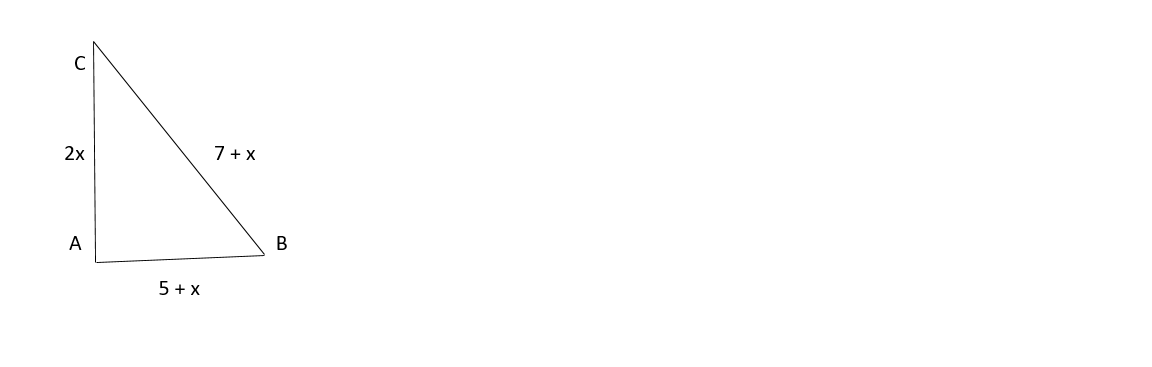Pythagoras Theorem Formula from the above diagram

${\mathrm{BC}}^{2}+{\mathrm{AB}}^{2}={\mathrm{AC}}^{2}$

BC = 7 + x , AB = 5 + x , AC = 2x

Substitute into the formula

${\mathrm{\left(7 + x\right)}}^{2}+{\mathrm{\left(5 + x\right)}}^{2}={\mathrm{\left(2x\right)}}^{2}$

Expand and simplify

$\mathrm{\left(7 + x\right)\left(7 + x\right)}+\mathrm{\left(5 + x\right)\left(5 + x\right)}=\mathrm{\left(2x\right)\left(2x\right)}$

$7×7+7×x+x×7+x×x=5×5+5×x+x×5+x×x+{\mathrm{4x}}^{2}$

$49 + 7x + 7x +{x}^{2}=25 + 5x + 5x +{x}^{2}+{\mathrm{4x}}^{2}$

Adding like terms on each side we have

$\mathrm{49}+\mathrm{14x}+{x}^{2}=\mathrm{25}+\mathrm{10x}+{\mathrm{5x}}^{2}$

Collecting like terms we have

$\mathrm{49 - 25 + 14x - 10x +}{x}^{2}-{\mathrm{5x}}^{2}$

$\mathrm{24}+\mathrm{4x}-{\mathrm{4x}}^{2}=0$

Rewriting the equation we have

${\mathrm{4x}}^{2}-\mathrm{4x}-\mathrm{24}=0$

From the above quadratic equation find the value of x by factorising

${\mathrm{4x}}^{2}-\mathrm{4x}-\mathrm{24}=0$

$\frac{{\mathrm{4x}}^{2}}{4}-\frac{\mathrm{4x}}{4}-\frac{\mathrm{24}}{4}=\frac{0}{4}$

${x}^{2}-x-6=0$

product = -6

Sum = -1

Factors = -3, 2

${x}^{2}+\left(\mathrm{2x}-\mathrm{3x}\right)-6=0$

${x}^{2}+\mathrm{2x}-\mathrm{3x}-6=0$

$x\left(\mathrm{x + 2}\right)-3\left(\mathrm{x + 2}\right)=0$

$\left(\mathrm{x + 2}\right)=0$ or $\left(\mathrm{x - 3}\right)=0$

$x=3$ or $x=\mathrm{-2}$

## Example 2

Let AB and BC be the slant height in a diagram below. Hence find the lenth BC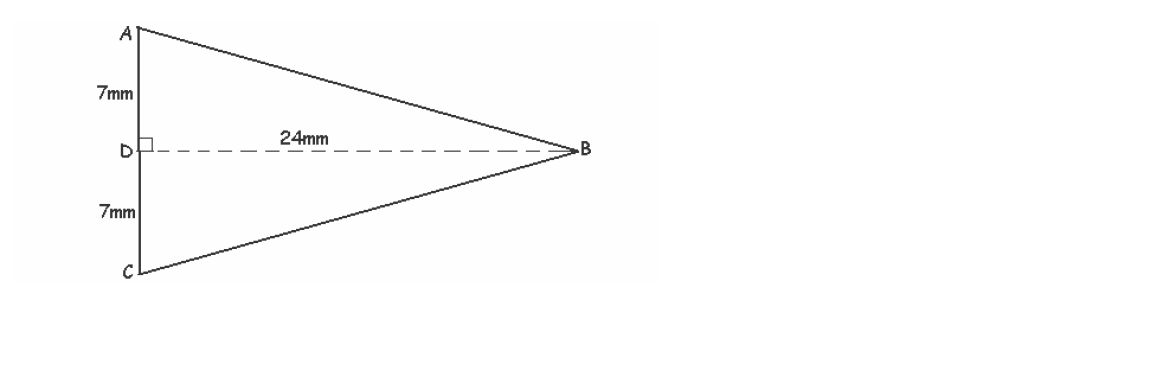${a}^{2}={b}^{2}+{c}^{2}$

Solutions:

${\mathrm{BC}}^{2}={\mathrm{BD}}^{2}+{\mathrm{DC}}^{2}$

Given that BC = ? , BD = 24mm and DC = 7mm

${\mathrm{BC}}^{2}={\mathrm{24}}^{2}+{7}^{2}$

${\mathrm{BC}}^{2}=\mathrm{24}+24+7+7$

${\mathrm{BC}}^{2}=\mathrm{576}+\mathrm{49}$

${\mathrm{BC}}^{2}=\mathrm{625}$

$\sqrt{\mathrm{BC}}=\sqrt{\mathrm{625}}$

Answers: $\mathrm{BC}=\mathrm{25mm}$

## Example 3

Calculate the distance x taken by a boy to move from the cliff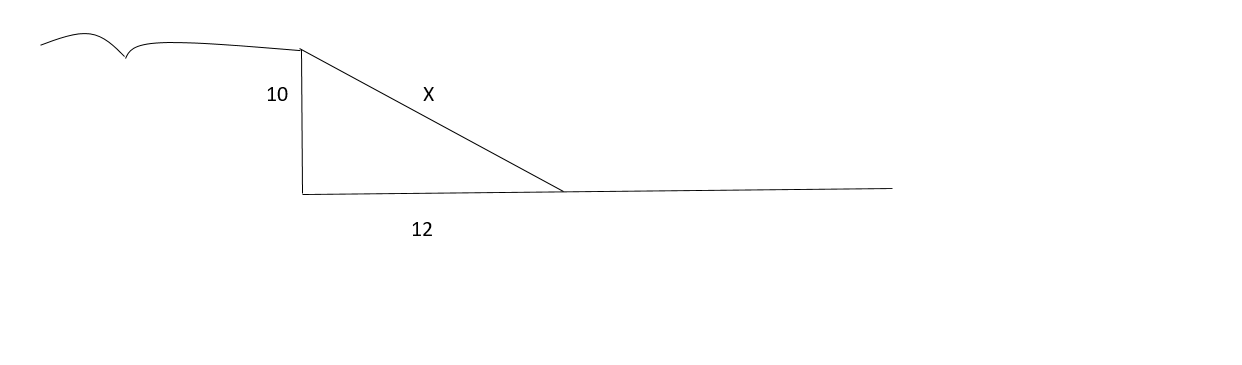${X}^{2}={\mathrm{10}}^{2}+{\mathrm{12}}^{2}$

${X}^{2}=100+144$

${X}^{2}=244$

$\sqrt{{X}^{2}}=\sqrt{244}$

$X=15.62$

## How to use pythagoras theorem

Two buildings are built next to each other and are joined by a footbrigdge of lenth 20 meters and the distance between than is 15 metres. Find the height h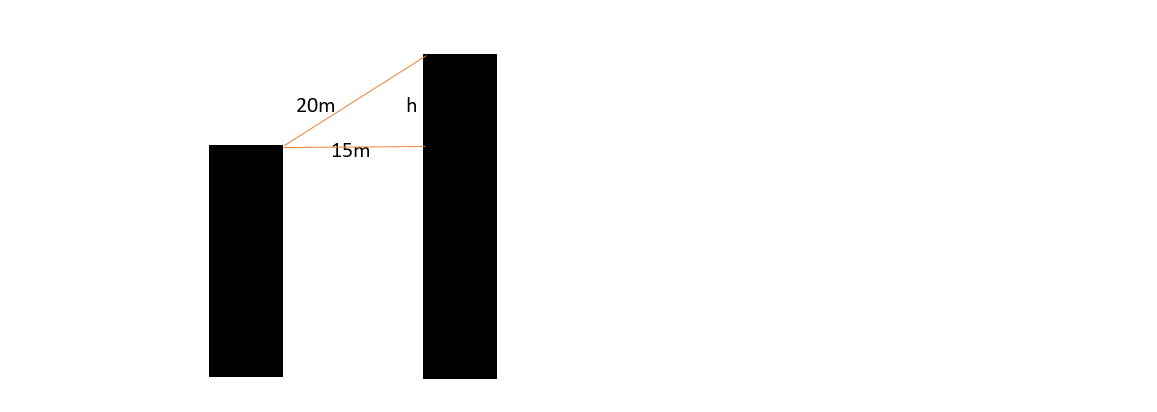${\mathrm{hypotenuse}}^{2}={\mathrm{adjacent}}^{2}+{\mathrm{opposite}}^{2}$

${\mathrm{20}}^{2}={\mathrm{15}}^{2}+{h}^{2}$

${h}^{2}={\mathrm{20}}^{2}-{\mathrm{15}}^{2}$

${h}^{2}=\mathrm{400}-225$

${h}^{2}=\mathrm{175}$

$\sqrt{{h}^{2}}=\sqrt{\mathrm{175}}$

$h=\mathrm{13.23 m}$

## How to solve Pythagoras Theorem

It takes 8 km for a drive to move from town B to town A and 6km from town A to town C , find the distance the drive will take to travel from time C to town B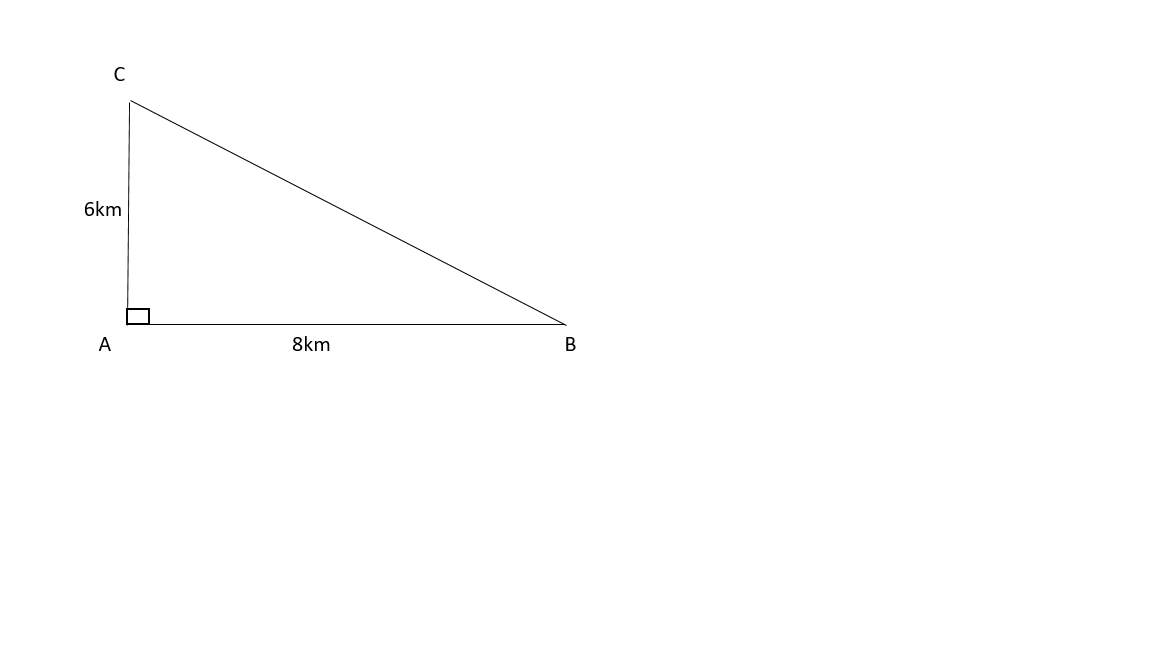$\sqrt{{\mathrm{BC}}^{2}}=\sqrt{{\mathrm{AB}}^{2}+{\mathrm{AC}}^{2}}$

$\mathrm{BC}=\sqrt{{\mathrm{AB}}^{2}+{\mathrm{AC}}^{2}}$

$\mathrm{BC}=\sqrt{{8}^{2}+{6}^{2}}$

$\mathrm{BC}=\sqrt{\mathrm{64}+\mathrm{36}}$

$\mathrm{BC}=\sqrt{\mathrm{100}}$

$\mathrm{BC}=\mathrm{10 km}$